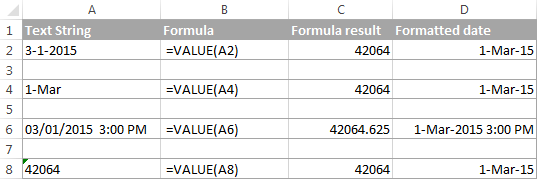# How to convert excel text to date in Excel

August 1, 2022
1.2K Views
0

A free Office suite fully compatible with Microsoft Office

A free Office suite fully compatible with Microsoft Office

In some cases, you can format the date and save it as text in a cell. For example, you may have entered a date in a cell formatted as text, or the data may have been imported or pasted as text from an external data source.

If you find text data in an Excel file, it is recommended that you convert these text strings to regular Excel data so that you can refer to them in formulas and perform various calculations. Also, as is often the case with Excel, there are several ways to tackle a task.

# Changing text to date by DATEVALUE

Excel's DATEVALUE function converts a date in text format to a serial number that Excel recognizes as a date.

1.   Excel's DATEVALUE syntax is very simple.

=DATEVALUE (date_text)

Therefore, the expression to convert a text value to a date is as simple as = DATEVALUE (A1). Where A1 is the cell where the date is stored as a text string.

2.   Excel's DATEVALUE function converts a date in text to a serial number, so you need to apply a date format to make that number look like a date, as explained earlier.

The following screenshot shows the behavior of some ExcelDATEVALUE formulas:ExcelVALUE Function-Converts a text string to a date

The ExcelVALUE function is more versatile than the DATEVALUE. You can convert text strings like dates and numbers to numbers. You can easily change the numbers to the date format of your choice. The syntax of the

VALUE function is: VALUE (text)

where text is a reference to a text string or cell that contains the text to convert to a number. The

Excel VALUE function can handle both date and time, the latter being converted to a fractional part, as shown in line 6 of the screenshot below:Text to date conversion using mathematics

You can force Excel to conduct a text-to-date conversion by performing basic math operations in addition to using specific Excel functions like VALUE and DATEVALUE. The operation must not alter the date's value in order to comply with the requirement (sequence number). Does it seem a little complicated? You can easily follow the example that follows! Given that the date in the text is in cell A1, one of the following formulas can be used to apply the date format to the cell:

1.   To add: = A1 + 0

2.   A1 multiplied by one is equal to

3.   Divide by A1 to get 1.

4.   The dual negation is =-A1.

Math operations may convert dates (lines 2 and 4), times (lines 6), and integers represented as text, as you can see in the screenshot above (lines 8). You don't need to bother about modifying the cell format because the outcome can be displayed as a date automatically.